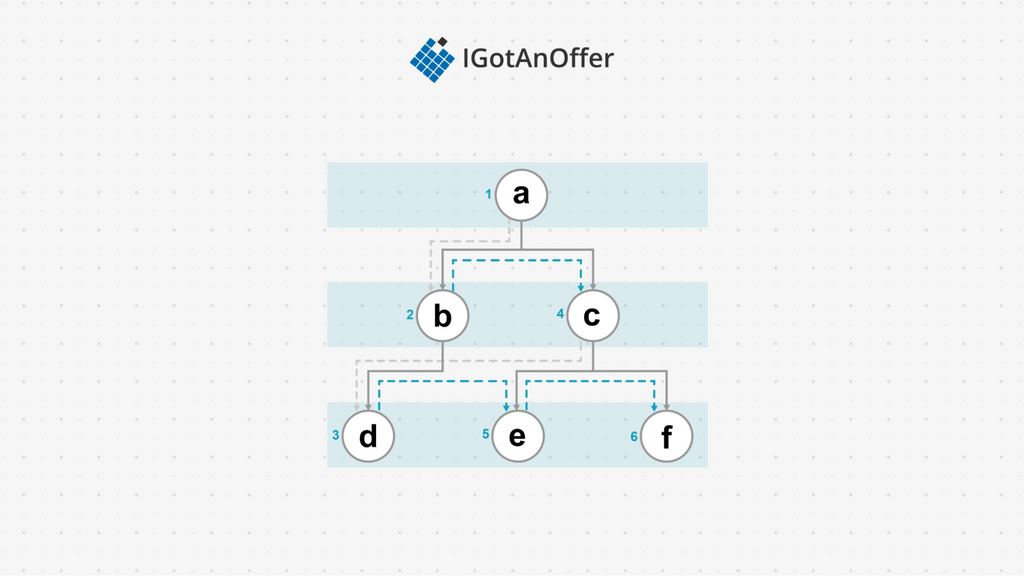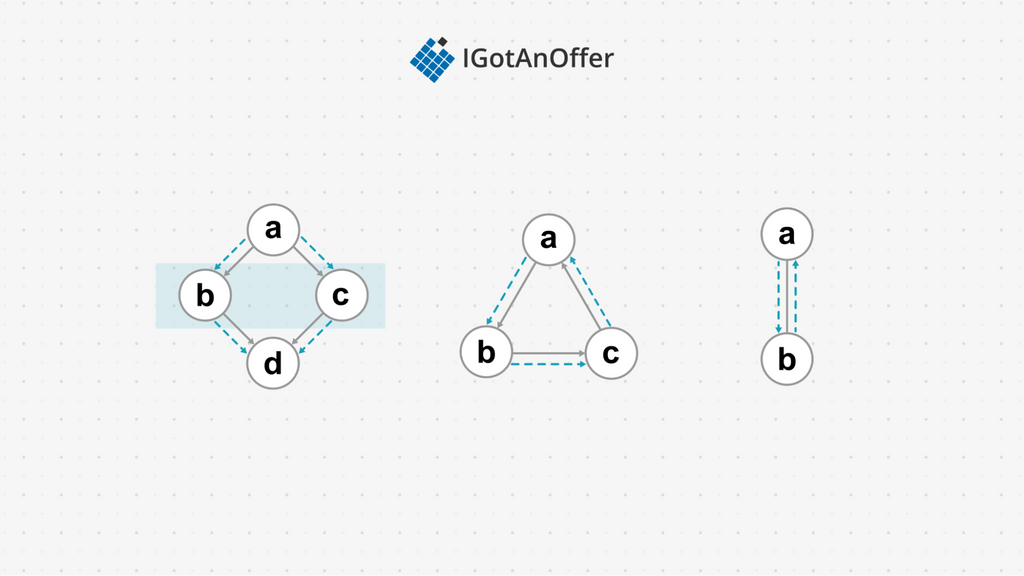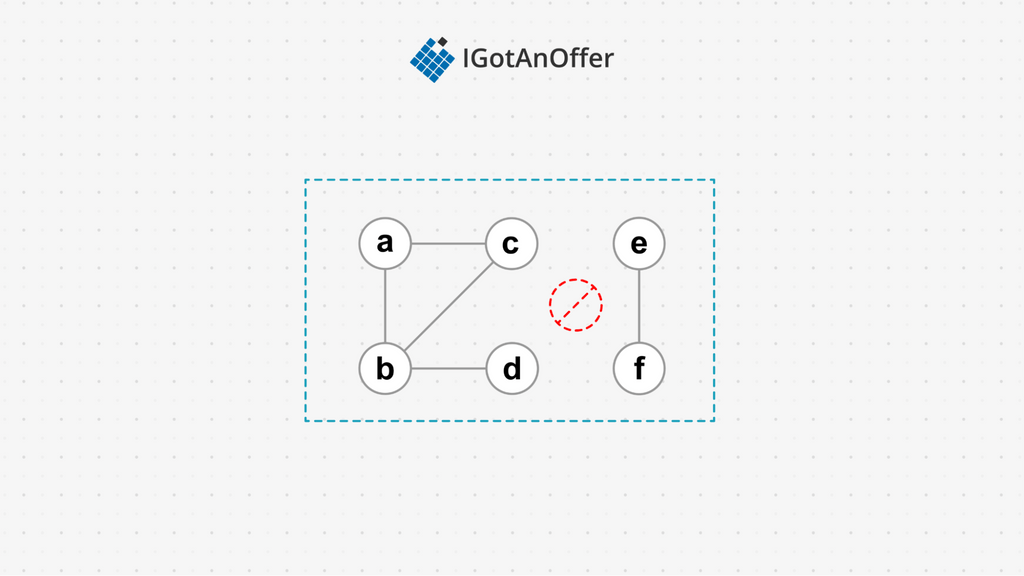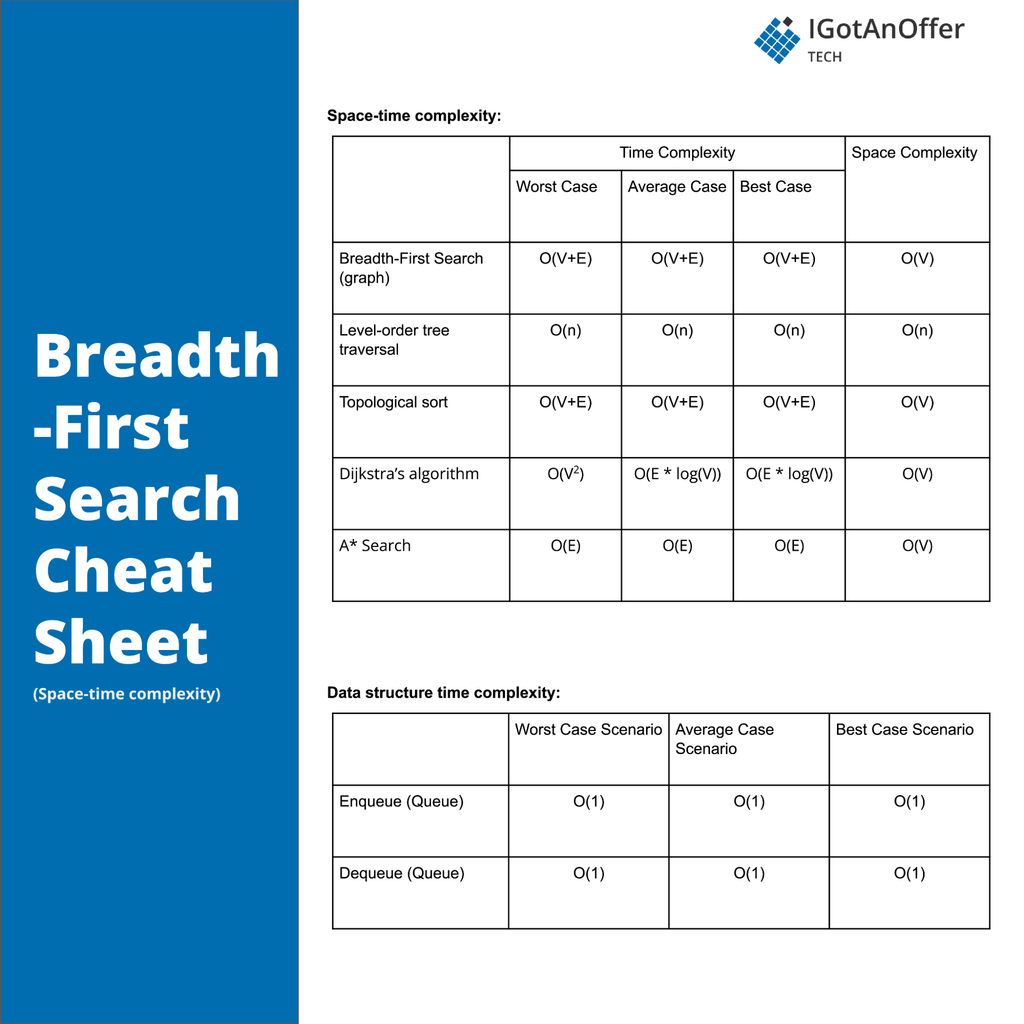# Breadth-first search (BFS): algorithms explained (2 of 8)

Breadth-first search is an important search algorithm for software engineers to know because it’s used as the foundation of many searching and optimization algorithms.

Below, we’ll explain exactly what breadth-first search is, how it works, and when and how to implement it. We’ve also included a handy cheat sheet so you can check its space-time complexity at a glance.

Here’s an outline of what we’ll cover:

Let's get into it.

## 1. Breadth-first search basics

In order to crack questions about breadth-first search, you’ll need to have a strong understanding of the algorithm, how it works, and when to use it.

### 1.1 What is breadth-first search?

We can categorize all data structures into two categories: those with a simple option for visiting each item, and those with multiple, complex options for visiting each item. Data structures like arrays, list, stacks, and queues fall in the first category – we can easily visit each node in an array by traversing over the array indices; following each link for a list; popping from a stack; and dequeuing from a queue. But for a tree or graph data structure, it is not so simple.

Breadth-first search (BFS) is one traversal method for trees and graphs in which all vertices on one layer are visited before visiting their children on the next layer – i.e. every node on layer i is visited before the nodes on layer i+1. In the following example, traversal starts at the root node a, moves to the nodes in the next layer (b and c), and finally visits the nodes in the last layer, giving us an output of abcdef.#### 1.1.1 Use cases for breadth-first search (Java, Python, C++)

This search type has many use cases, some of which are:

• Finding the quickest or shortest solution to a problem. For example, in the tree above, if we start at state a and have states c and d as goal nodes, then BFS will stop once c is found. This is the optimal solution, as it is one move away from a while d is two moves away
• Implementing a crawler (like a web scraper) that stops once an arbitrary depth has been reached
• Detecting cycles in graphs
• Establishing whether a graph is connected (any node can be reached from any other) or disconnected (there are "islands" that cannot be reached from other parts of the graph)
• Checking if people in a social network are connected within k degrees of separation

BFS is also used in other algorithms, such as minimum spanning trees, Dijkstra’s shortest path algorithm, the Ford-Fulkerson algorithm, and Cuthill-McKee’s algorithm.

#### 1.1.2 Example implementation

Let’s consider a BFS implementation for searching a tree data structure. At a high level, we need to keep track of the next layer of nodes to visit. This is done by pushing the current node’s children to a queue. In other words, child nodes on each level are visited after all parent nodes have been visited.

This tree algorithm will not work for graphs, since graph vertices can have more than one edge pointing to them. Take a look at the following three graphs.In the first graph, the algorithm visits a, followed by its children, b and c. On visiting b and c, d will be pushed to the queue, resulting in d being visited twice. In the second graph, the algorithm will visit abc in an infinite cycle. In the last undirected graph, ab will be visited repeatedly.

The solution is to keep track of the vertices as they are visited. Here is a revised implementation for graphs that introduces the visited set:

This algorithm solves the previous three issues. In the first graph, expanding c won’t push d, since d is already in the visited set. In the second graph, expanding c won’t push a, since a is already in the visited set. Similarly in the third graph, the algorithm will not push a to the queue on visiting b, as a will be in the visited set.

A final case to consider is a disconnected graph, like the one below, in which all vertices need to be visited.If our algorithm begins its search on the e vertex of the right subgraph, it will push f to the queue and stop. The left subgraph will not be visited. Starting with any of the vertices on the left subgraph would have the same result: the algorithm would not visit any of the vertices on the right.

We can solve this with the following revision:

Here we start the algorithm for every possible vertex in the graph. A loop is added to kickoff the algorithm for every vertex.

#### 1.1.3 How breadth-first search compares to other algorithms

One alternative to BFS is depth-first search, but BFS is complete. This means that if the search space is infinite, then BFS will find the solution since BFS searches each layer at a time. In an infinite space, depth-first search can get trapped and will never find the solution, but in many cases DFS can find the solution faster if it exists in one of the paths explored first.

An adaptation of depth-first search called iterative deepening search (IDS) can be seen as a hybrid between BFS and depth-first search. IDS provides the completeness of BFS, with the advantages of depth-first search.

BFS assumes each edge of a vertex has an equal cost (an implicit cost of 1), but this might not always be the case. Consider distances between cities, for example. Uniform-cost search is an algorithm that uses a priority queue to find the next node to expand.

## 2. Breadth-first search cheat sheetYou can download the cheat sheet here.

### 2.2 Cheat sheet explained

The cheat sheet above is a summary of information you might need to know for an interview, but it’s usually not enough to simply memorize it. Instead, aim to understand each result so that you can give the answer in context.

The cheat sheet is broken into time complexity and space complexity (the processing time and memory requirements for breadth-first search). Breadth-first search is related to several data structures, which are also included in the cheat sheet.

For more information about time and space requirements of different algorithms, read our complete guide to big-O notation and complexity analysis.

#### 2.2.1 Time complexity

The tree implementation of BFS visits each node in the tree for a complexity of O(n). A stop condition can stop the search earlier and will, on average, visit half the nodes. So the worst and average case will be O(n).

The graph implementation of BFS visits each vertex and edge for a complexity of O(V + E). Again, a stop condition might stop the search early, visiting half the vertices on average. So the worst and average case will be O(V + E).

#### 2.2.2 Space complexity

For the tree implementation of BFS, the queue size will be bounded by the number of leaf nodes. On average, this will be half of all the nodes, but it could be all the nodes except the root, for example, for a tree with one root node and all other nodes being direct children of the root. In both cases BFS has a space complexity of O(n).

For the graph implementation of BFS, every vertex will be in the visited set at the end of the search, giving a space complexity of O(V).

#### 2.2.3 Related algorithms and data structures: Dijkstra’s algorithm

Dijkstra’s shortest path algorithm is an adaptation of BFS, in which each edge has a weight. Rather than taking the oldest item of the queue (FIFO), it uses a priority queue to dequeue the node with the shortest distance from those nodes to visit next. When this node is expanded, its weight is added to the distance to its children before each child is enqueued. This can then be used to find the shortest route between two cities on a map, for example. The algorithm will stop when the destination vertex (the destination city, in our example) is dequeued.

Like a graph BFS, each vertex and edge will be visited in the worst case, and only half in the average case. Dequeuing from a priority queue is constant time. Combined, these give both cases a time complexity of O(V + E). For space complexity, all the vertices might end up in the visited set resulting in O(V).

When all the edges in the graph have an equal weight, Dijkstra’s shortest path will reduce to the first graph implementation for BFS.

## 3. How to prepare for a coding interview

### 3.1 Refresh your knowledge

Before you start practicing interviews, you’ll want to make sure you have a strong understanding of not only breadth-first search but also the rest of the relevant algorithms and data structures. Check out our guides for detailed explanations and useful cheat sheets.

Algorithms explained:

Data structures explained:

### 3.2 Practice on your own

Once you’re confident in all of these, you’ll want to start working through lots of coding problems.

Check out the articles in our algorithms questions series for practice on the most important algorithms.

We also recommend working through our list of 73 data structure interview questions.

One of the main challenges of coding interviews is that you have to communicate what you are doing as you are doing it. Talking through your solution out loud is therefore very helpful. This may sound strange to do on your own, but it will significantly improve the way you communicate your answers during an interview.

It's also a good idea to work on a piece of paper or google doc, given that in the real interview you'll have to code on a whiteboard or virtual equivalent.

### 3.3 Practice with others

Of course, if you can practice with someone else playing the role of the interviewer, that's really helpful. But sooner or later you’re probably going to want some expert interventions and feedback to really improve your coding interview skills.

That’s why we recommend practicing with ex-interviewers from top tech companies. If you know a software engineer who has experience running interviews at a big tech company, then that's fantastic. But for most of us, it's tough to find the right connections to make this happen. And it might also be difficult to practice multiple hours with that person unless you know them really well.

Here's the good news. We've already made the connections for you. We’ve created a coaching service where you can practice 1-on-1 with ex-interviewers from leading tech companies. Learn more and start scheduling sessions today.